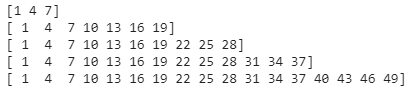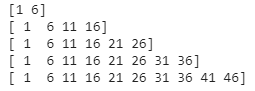Related Articles

# How to Create a Sequence of Linearly Increasing Values with Numpy Arrange?

• Last Updated : 05 Aug, 2021

In this article, we are going to create a sequence of linearly increasing values with Numpy arrange() function.

### Getting Started

By using arrange() function, we can create a sequence of linearly increasing values. This method returns an array with evenly spaced elements as per the interval. The interval mentioned is half opened i.e. [Start, Stop)

Syntax: numpy.arrange(start, stop, step)

Parameters:

• start is the starting value
• stop is the ending value
• step is a linear increment value with in the given range. It is optional. By default, it is 1.

Return Type:

• Return an array of elements

For example:

`numpy.arrange(1,10,3)  # array([1,4,7])`

Here, we have given a range from 1 to 10(start = 1 and stop = 10) but we specified step=3. That means it skips every 3 elements in a range. So In this way, we can increase linearity in data.

Example 1:

Create elements within range with 3-step linearly.

## Python3

 `# importing numpy module``import` `numpy as np` `# create an elements from 1``# to 10 with 3 step linearity``print``(np.arrange(``1``, ``10``, ``3``))` `# create an elements from 1``# to 20 with 3 step linearity``print``(np.arrange(``1``, ``20``, ``3``))` `# create an elements from 1``# to 30 with 3 step linearity``print``(np.arrange(``1``, ``30``, ``3``))` `# create an elements from 1``# to 40 with 3 step linearity``print``(np.arrange(``1``, ``40``, ``3``))` `# create an elements from 1``# to 50 with 3 step linearity``print``(np.arrange(``1``, ``50``, ``3``))`

Output:Example 2:

Create elements within range with 5-step linearly.

## Python3

 `#importing numpy module``import` `numpy as np` `#create an elements from 1 to``# 10 with 5 step linearity``print``(np.arrange(``1``,``10``,``5``))` `#create an elements from 1 to``# 20 with 5 step linearity``print``(np.arrange(``1``,``20``,``5``))` `#create an elements from 1 to``# 30 with 5 step linearity``print``(np.arrange(``1``,``30``,``5``))` `#create an elements from 1 to``# 40 with 5 step linearity``print``(np.arrange(``1``,``40``,``5``))` `#create an elements from 1 to``# 50 with 5 step linearity``print``(np.arrange(``1``,``50``,``5``))`

Output:Example 3:

Create elements within the range of 34 to 50 with 5-step linearly.

## Python3

 `#importing numpy module``import` `numpy as np` `#create an elements from 34 to 50 with 4 step linearity``print``(np.arrange(``34``,``50``,``5``))`

Output:

`[34 39 44 49]`

Attention geek! Strengthen your foundations with the Python Programming Foundation Course and learn the basics.

To begin with, your interview preparations Enhance your Data Structures concepts with the Python DS Course. And to begin with your Machine Learning Journey, join the Machine Learning – Basic Level Course

My Personal Notes arrow_drop_up